Machine Learning theory and applications using Octave or Python.

## 1. Large Margin Classification

I would like to give full credits to the respective authors as these are my personal python notebooks taken from deep learning courses from Andrew Ng, Data School and Udemy :) This is a simple python notebook hosted generously through Github Pages that is on my main personal notes repository on https://github.com/ritchieng/ritchieng.github.io. They are meant for my personal review but I have open-source my repository of personal notes as a lot of people found it useful.

### 1a. Optimization Objective

• So far we have seen mainly 2 algorithms, logistic regression and neural networks. There are more important aspects of machine learning:
• The amount of training data
• Skill of applying the algorithms
• The SVM sometimes give a cleaner and more powerful way to learn parameters
• This is the last supervised learning algorithm in this introduction to machine learning
• Alternative view of logistic regression
• If we want hθ = 1, we need z » 0
• If we want hθ = 0, we need z « 0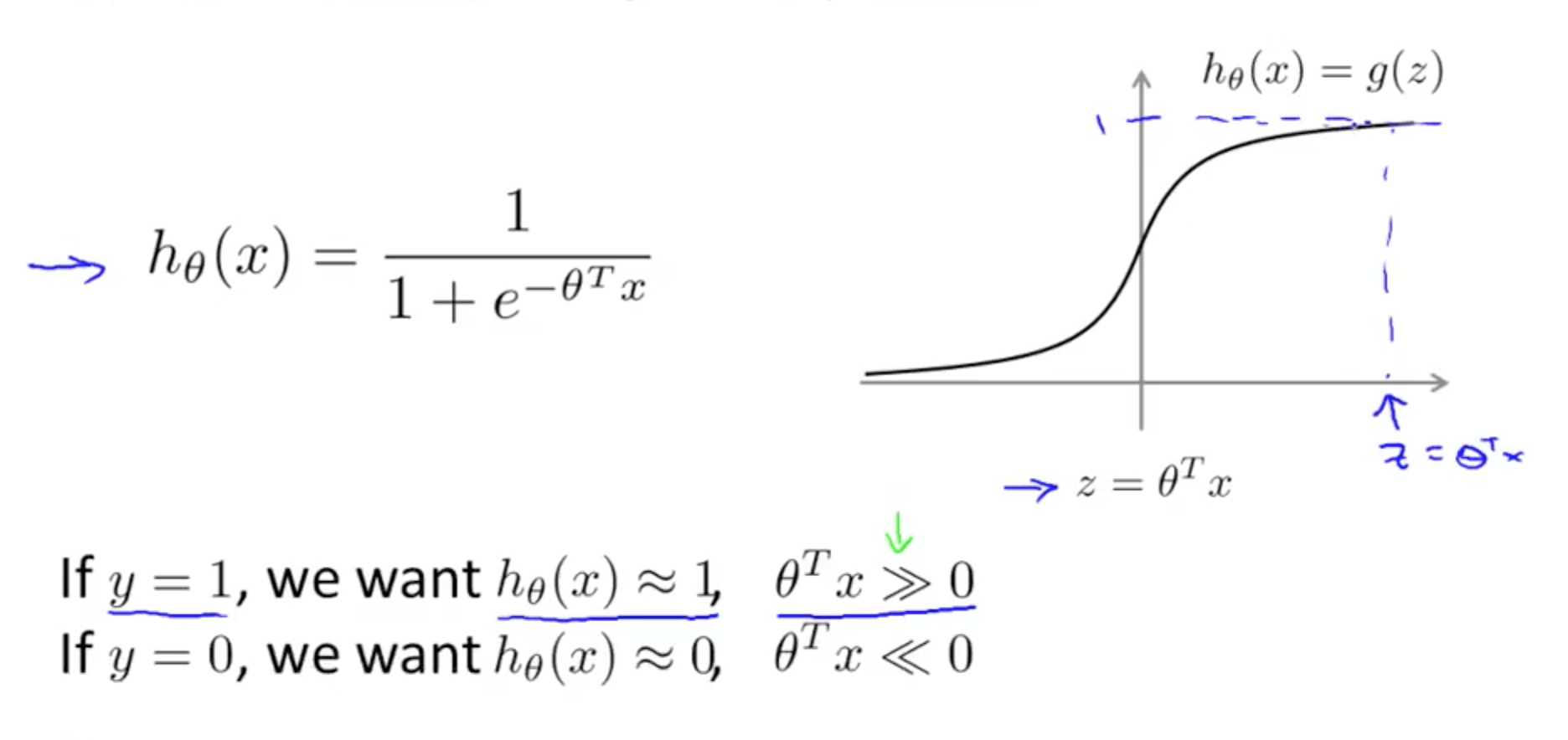• If y = 1, only the first term would matter
• Graph on the left
• When z is large, cost function would be small
• Magenta curve is a close approximation of the log cost function
• If y = 0, only the second term would matter
• Magenta curve is a close approximation of the log cost function
• Diagram of cost contributions (y-axis)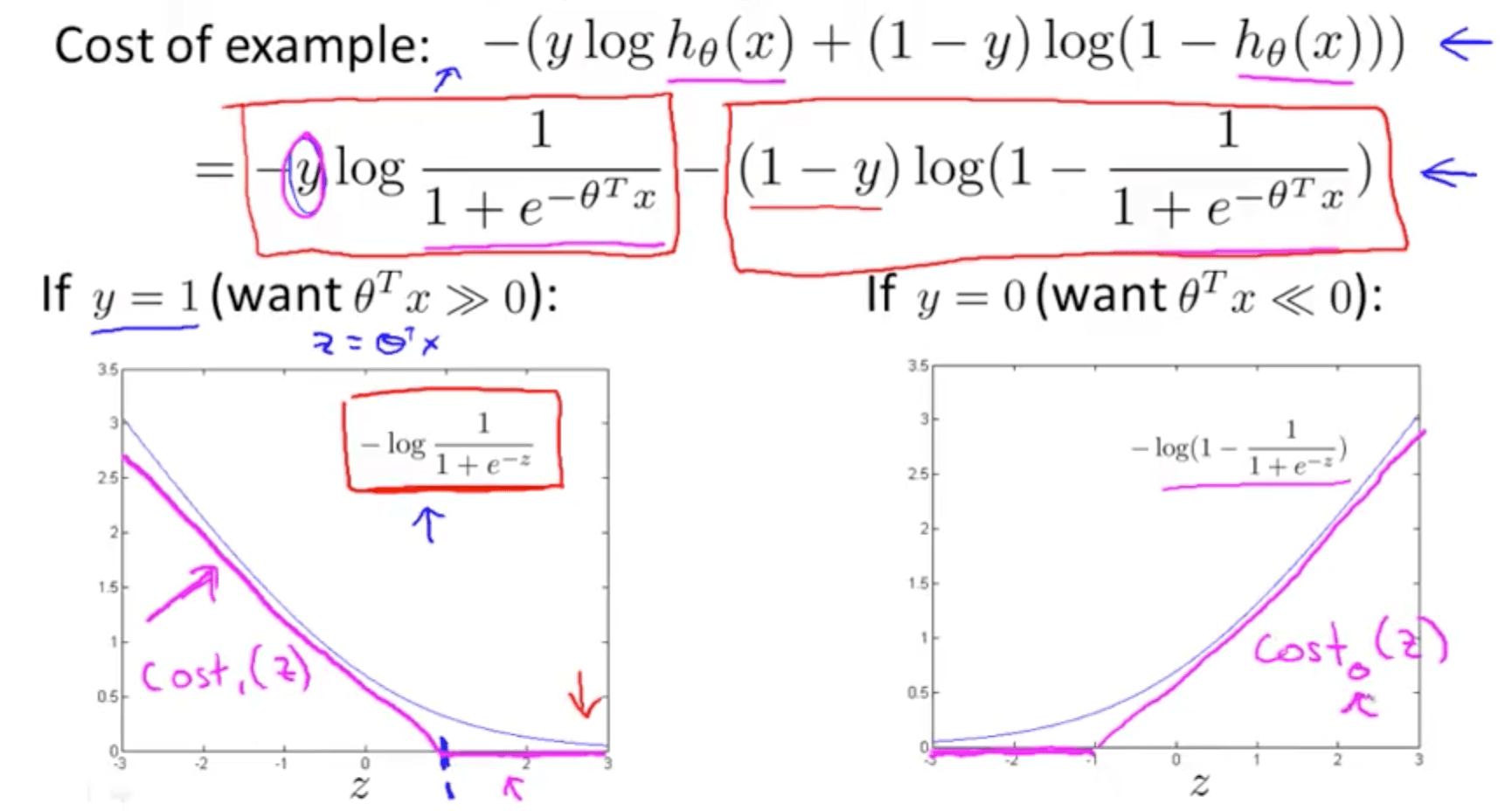• Support Vector Machine
• Changes to logistic regression equation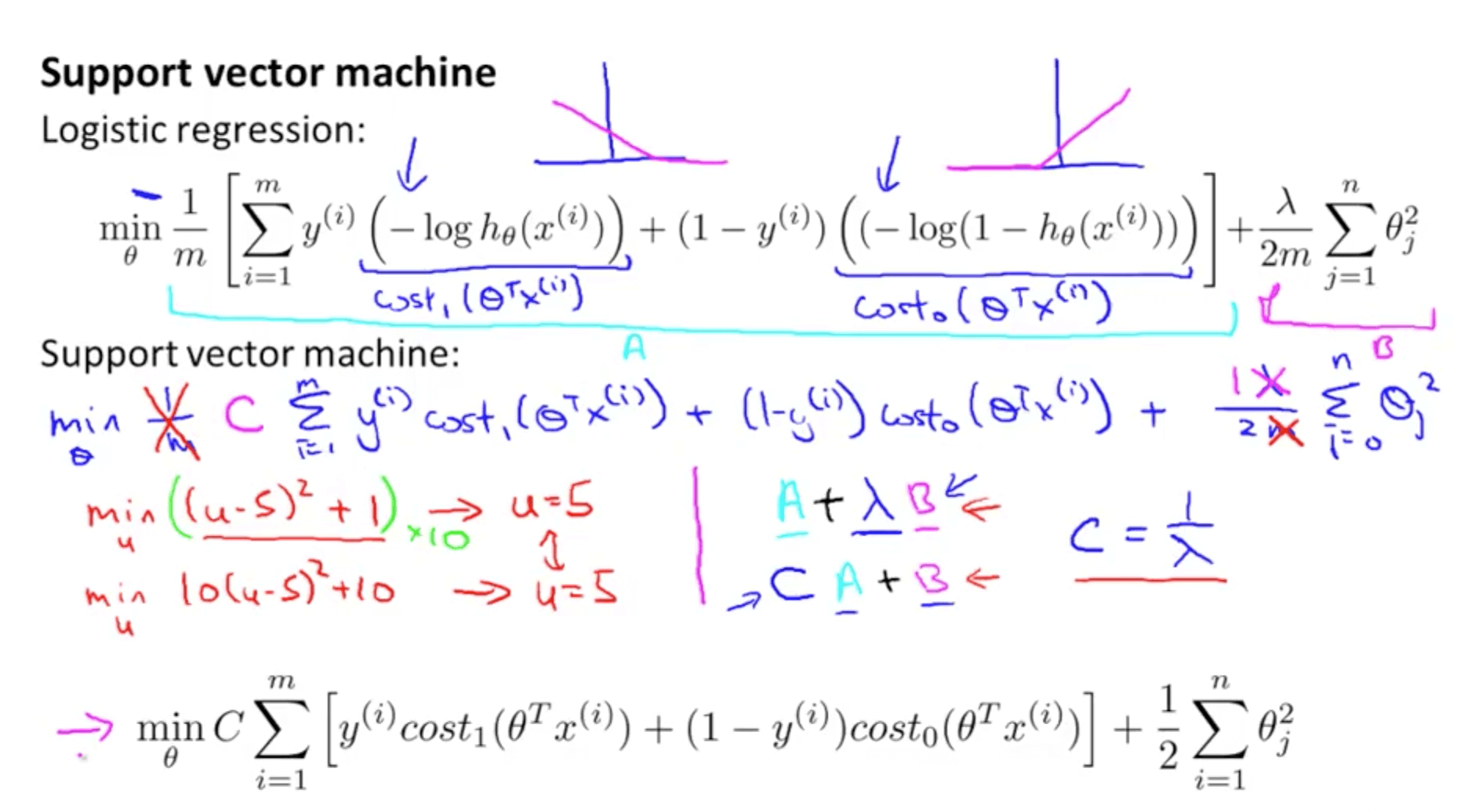• We replace the first and second terms of logistic regression with the respective cost functions
• We remove (1 / m) because it does not matter
• Instead of A + λB, we use CA + B
• Parameter C similar to the role (1 / λ)
• When C = (1 / λ), the two optimization equations would give same parameters θ
• Compared to logistic regression, it does not output a probability
• We get a direct prediction of 1 or 0 instead
• If θTx is => 0
• hθ(x) = 1
• If θTx is <= 0
• hθ(x) = 0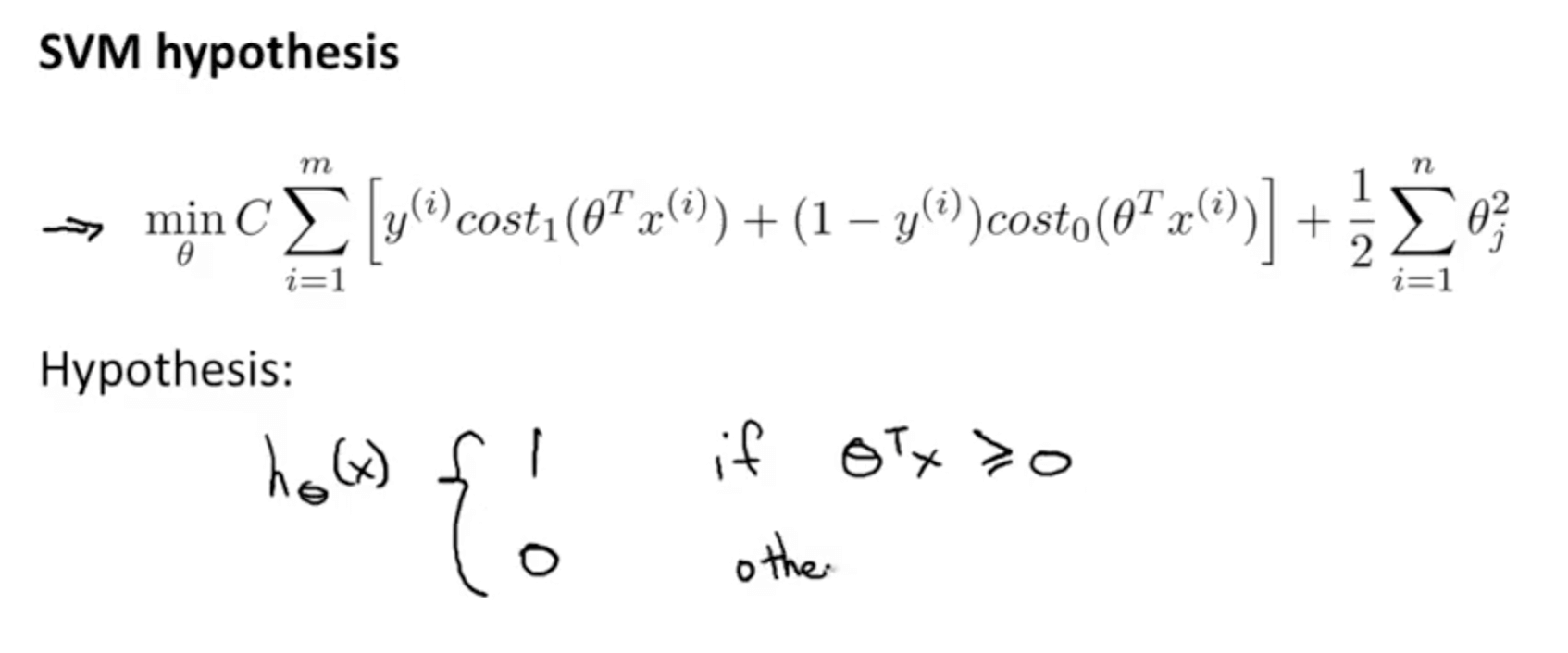### 1b. Large Margin Intuition

• Some times people call Support Vector Machines “Large Margin Classifiers”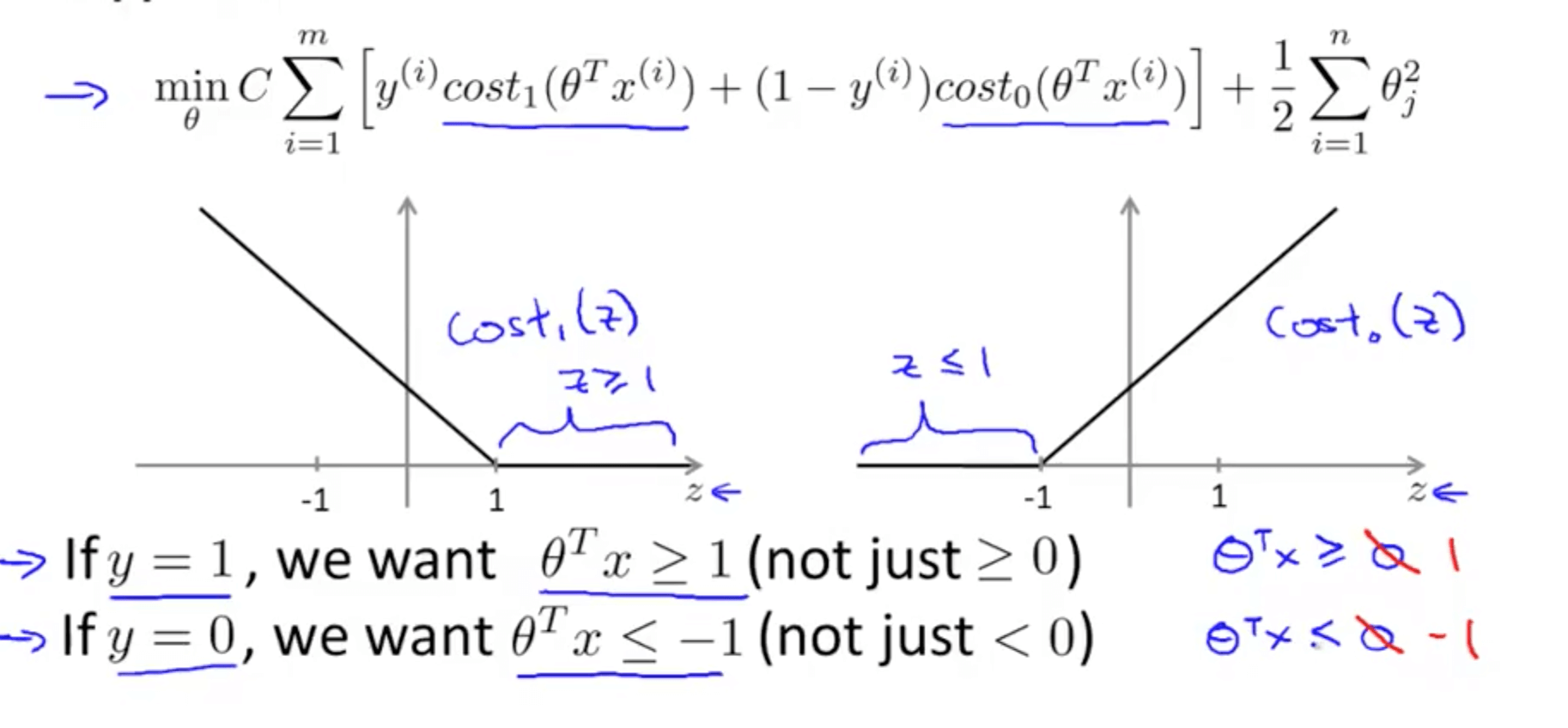• SVM decision boundary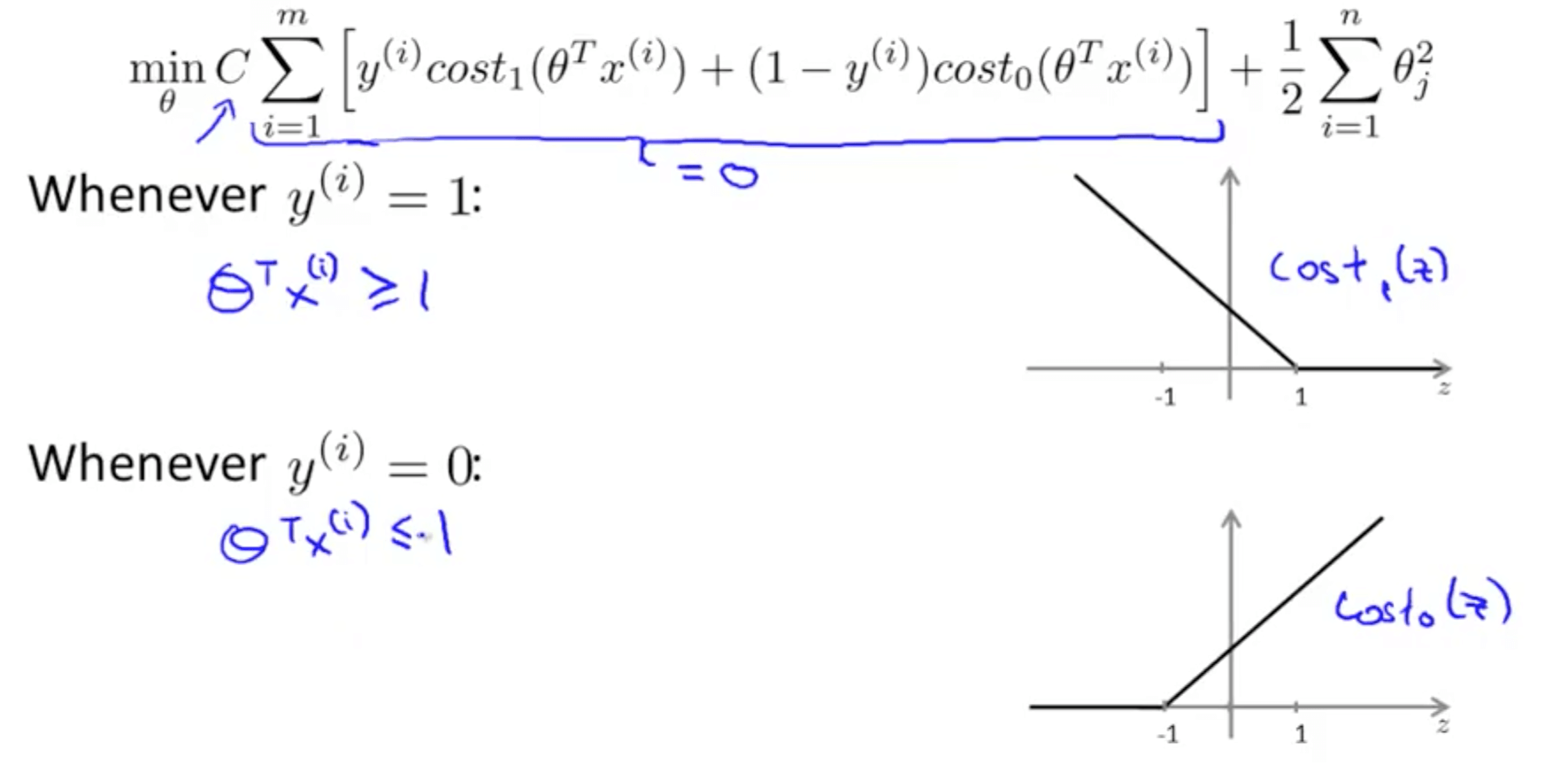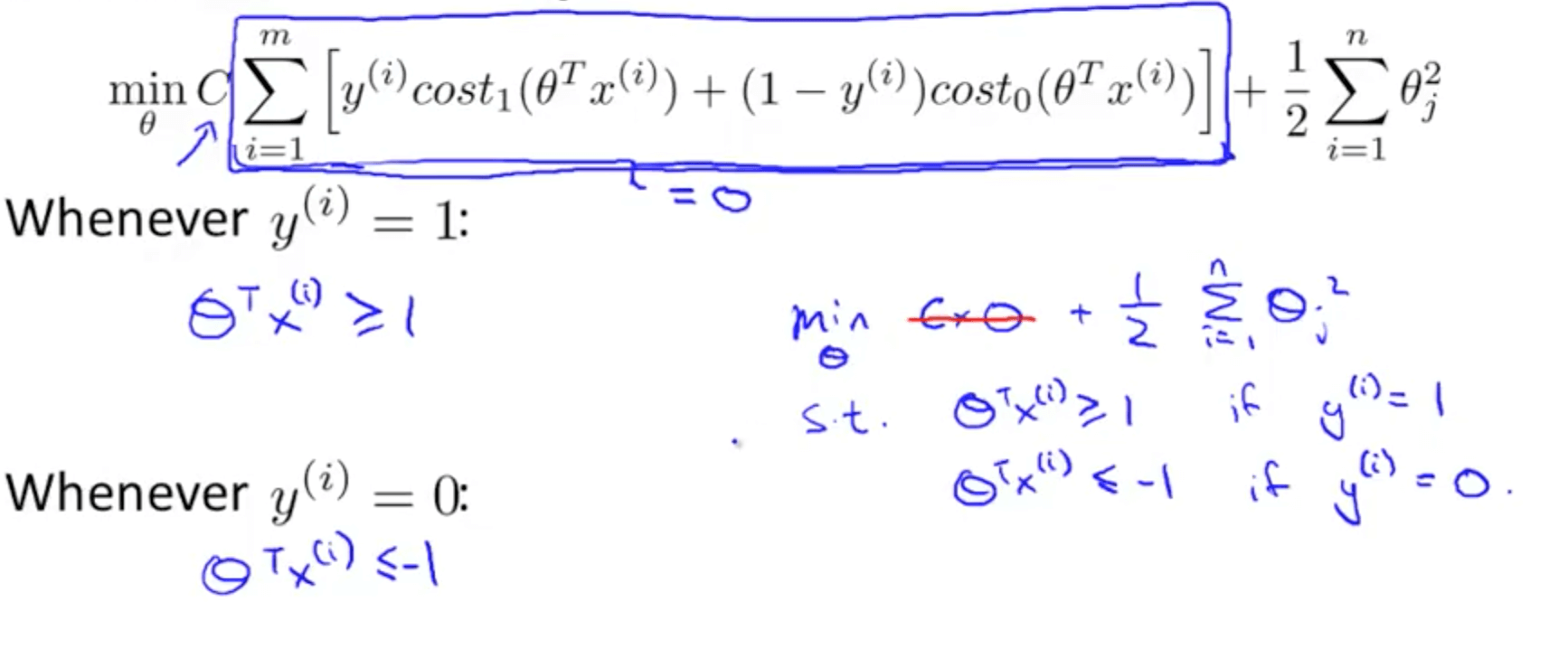• If C is huge, we would want A = 0 to minimize the cost function
• How do we make A = 0
• If y = 1
• A = 0 such that θTx >= 1
• If y = 0
• A = 0 such that θTx <= -1
• Since we want to ensure A = 0, our optimization problem boils down to minimizing the later term only
• SVM decision boundary: linearly separable case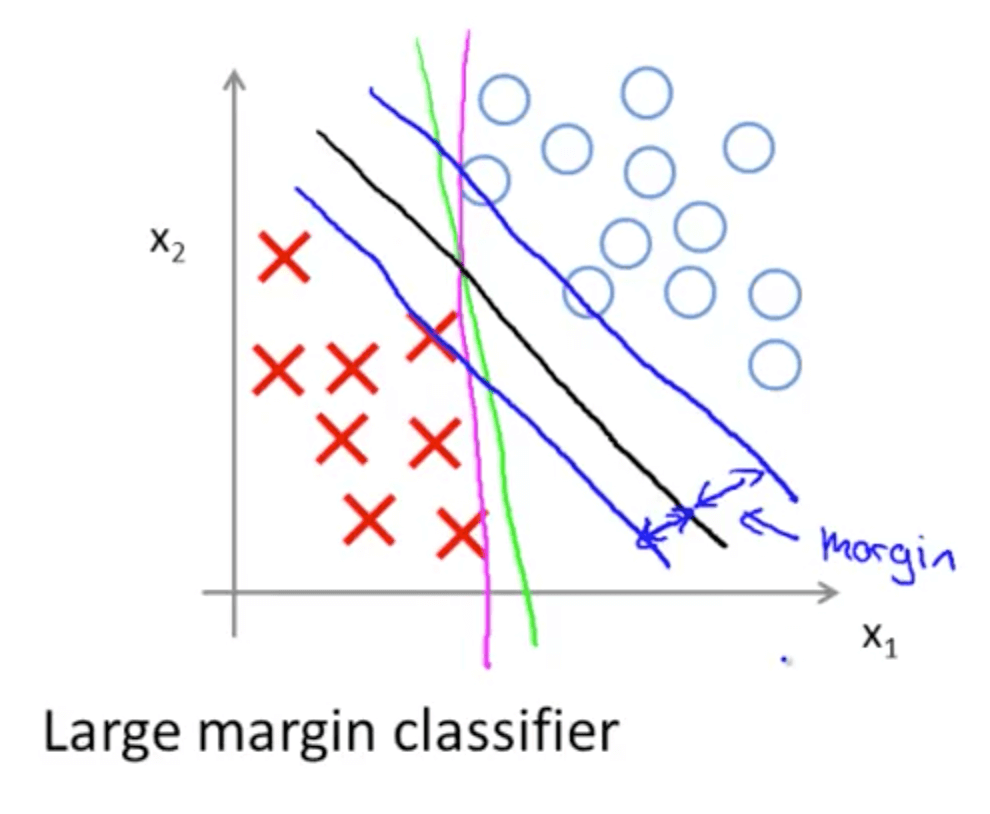• Black decision boundary
• There is a larger minimum difference
• Chosen by SVM because of the large margins between the line and the examples
• Magenta and green boundaries
• Close to examples
• Distance between blue and black line: margin
• If C is very large
• Decision boundary would change from black to magenta line
• If C is not very large
• Decision boundary would be the black line
• SVM being a large margin classifier is only relevant when you have no outliers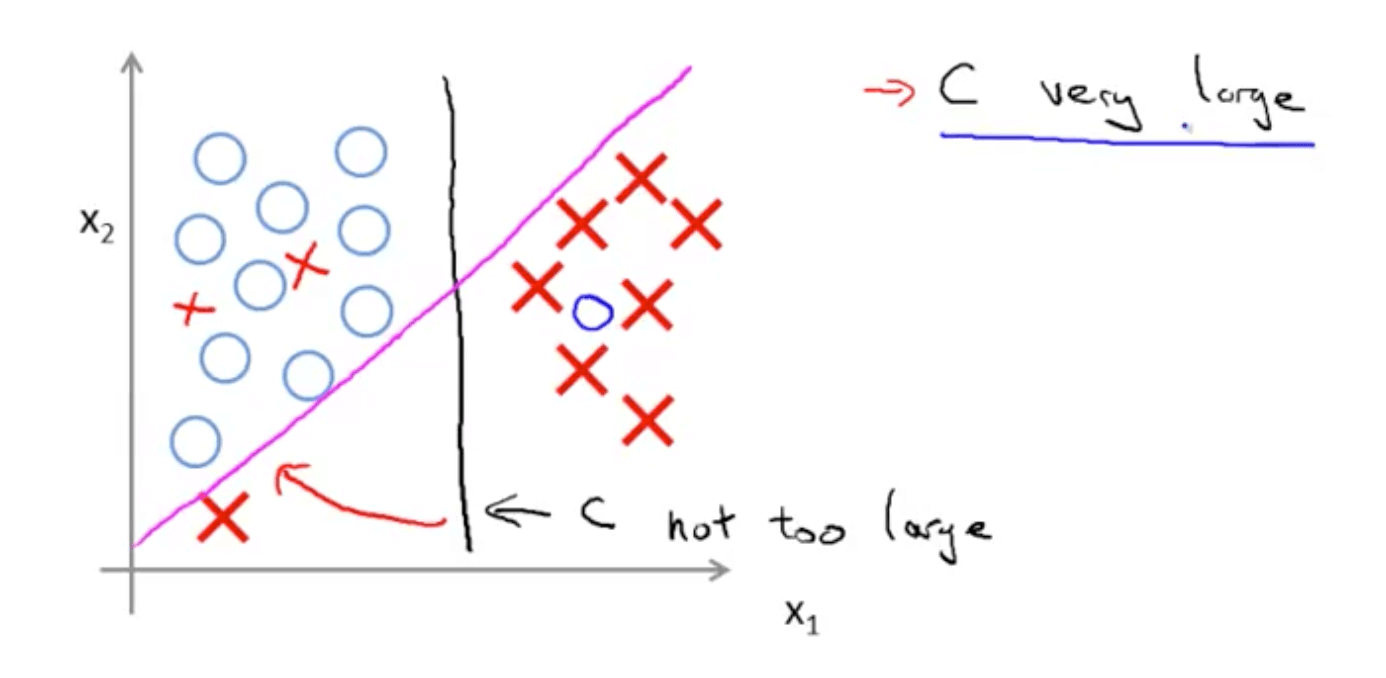### 1c. Mathematics of Large Margin Classification

• Vector inner product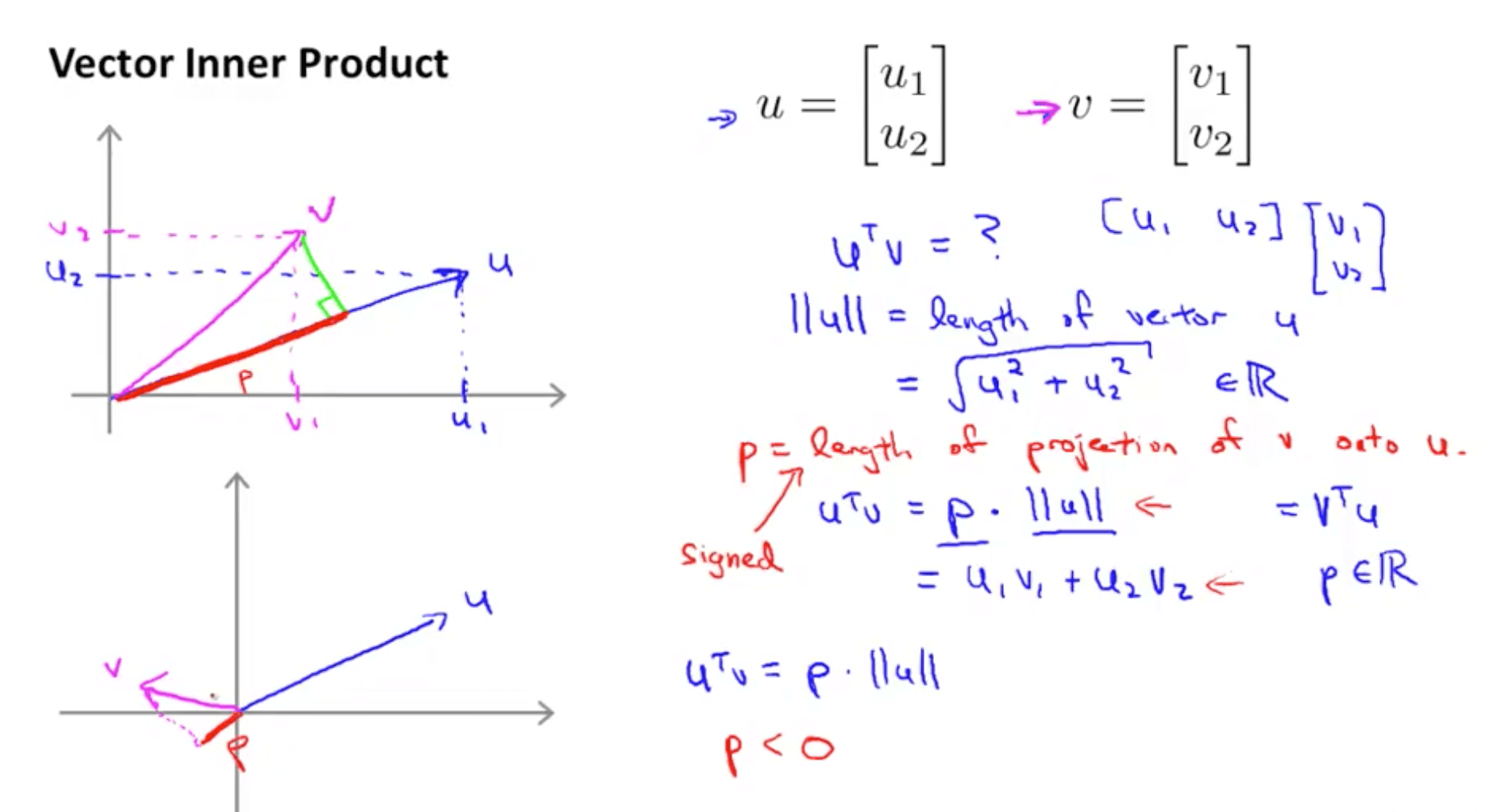• Brief details
• u_transpose * v is also called inner product
• length of u = hypotenuse calculated using Pythagoras’ Theorem
• If we project vector v on vector u (green line)
• p = length of vector v onto u
• p can be positive or negative
• p would be negative when angle between v and u more than 90
• p would be positive when angle between v and u is less than 90
• u_transpose * v = p . ll u ll = u1 v1 + u2 v2 = v_transpose * v
• SVM decision boundary: introduction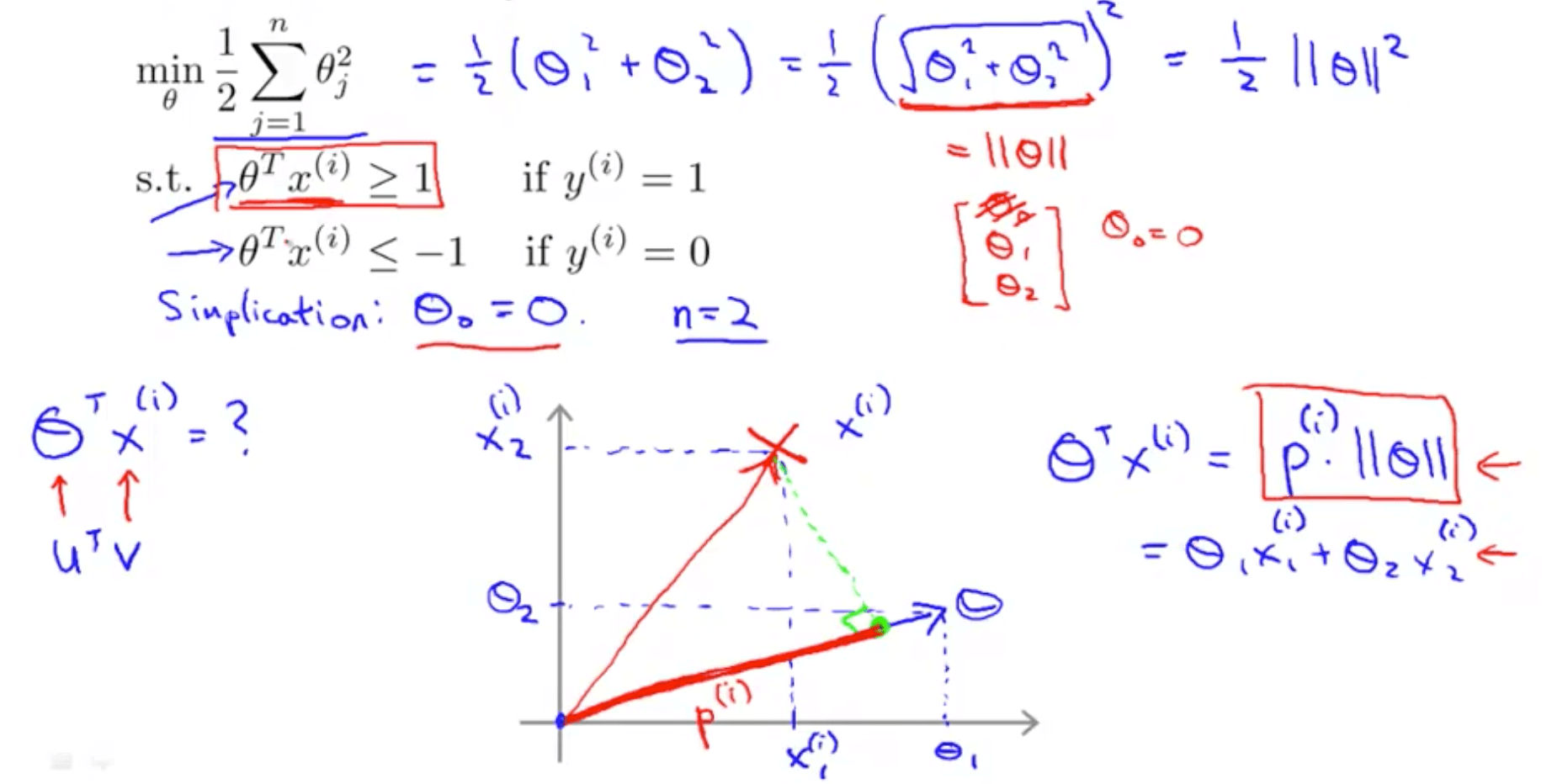• We set the number of features, n, to 2
• As you can see that normalization in SVM is minimizing the squared norm of the square length of the parameter θ, ll θ ll^2
• SVM decision boundary: projections and hypothesis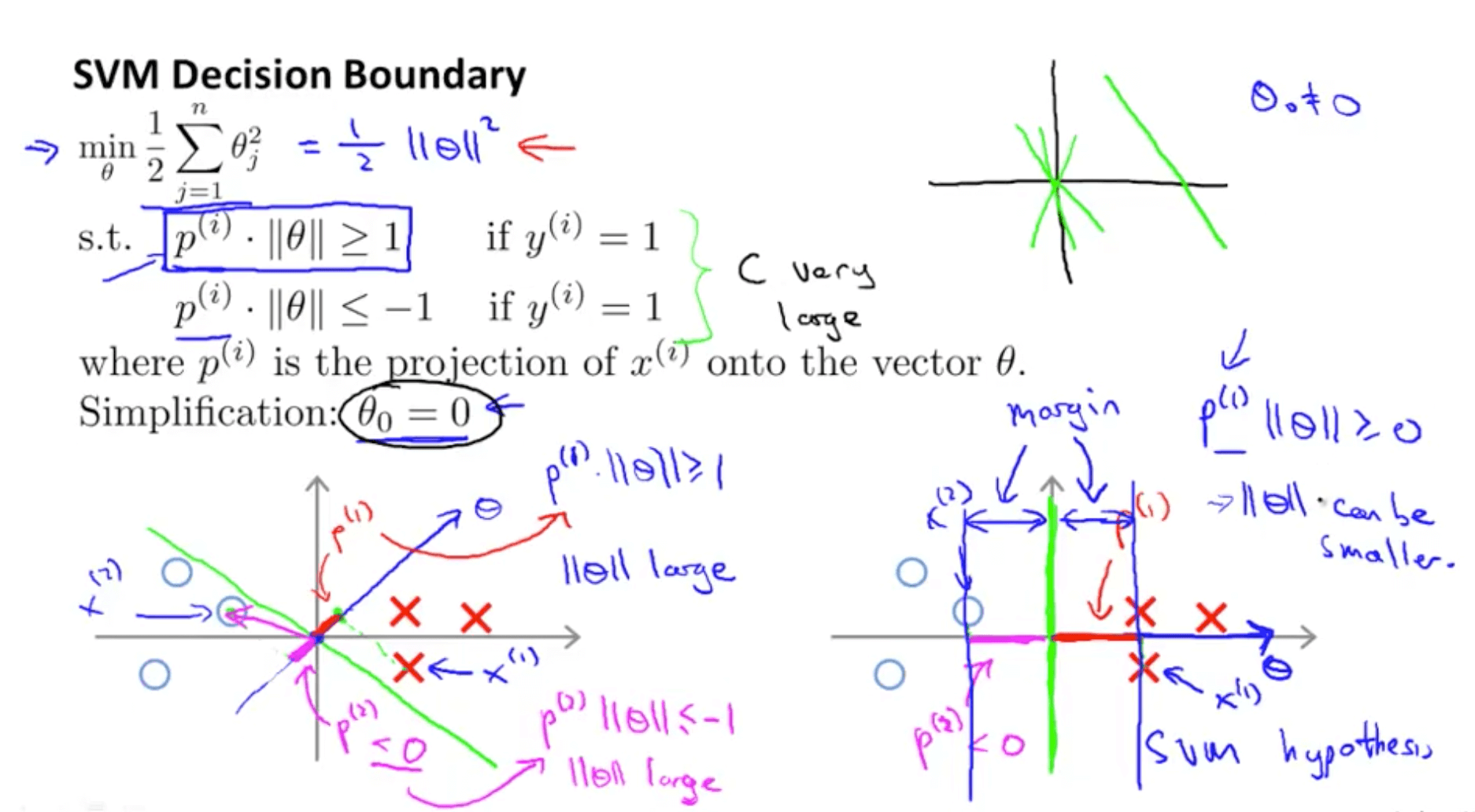• When θ0 = 0, this means the vector passes through the origin
• θ projection will always be 90 degrees to the decision boundary
• Decision boundary choice 1: graph on the left
• p1 is projection of x1 example on θ (red)
• p1 . ll θ ll >= 1
• For this to be true ll θ ll has to be large
• p2 is a projection of x2 example on θ (magenta)
• p2 . ll θ ll <= -1
• For this to be true ll θ ll has to be large
• But our purpose is to minimise ll θ ll^2
• This decision boundary choice does not appear to be suitable
• Decision boundary choice2: graph on the right
• p1 is projection of x1 example on θ (red)
• p1 is much bigger so norm of θ, ll θ ll, can be smaller
• p2 is a projection of x2 example on θ (magenta)
• p2 is much bigger so norm of θ, ll θ ll, can be smaller
• Hence ll θ ll^2 would be smaller
• And this is why SVM would choose this decision boundary
• Magnitude of margin is value of p1, p2, p3 and so on
• SVM would end up with a large margin because it tries to maximize the margin to minimize the squared norm of θ, ll θ ll^2

## 2. Kernels

### 2a. Kernels I

• Non-linear decision boundary
• Given the data, is there a different or better choice of the features f1, f2, f3 … fn?
• We also see that using high order polynomials is computationally expensive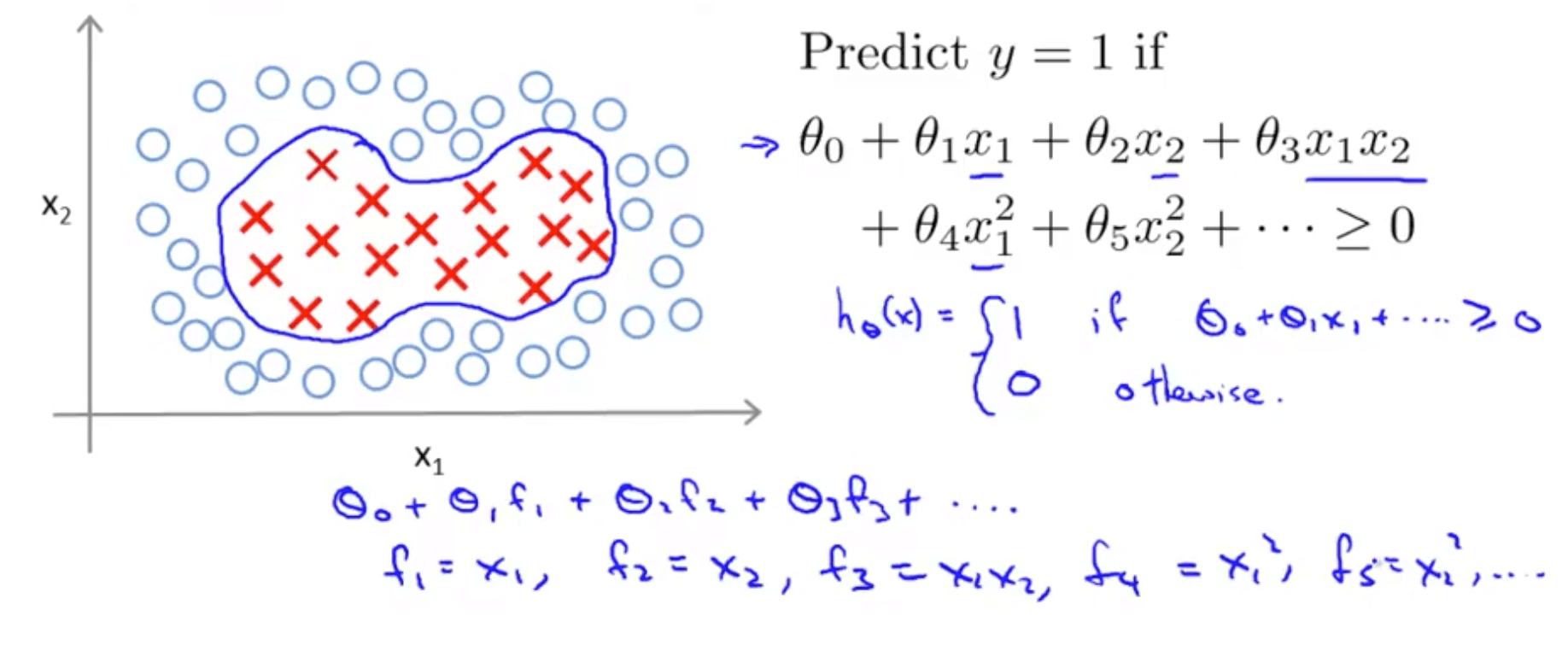• Gaussian kernel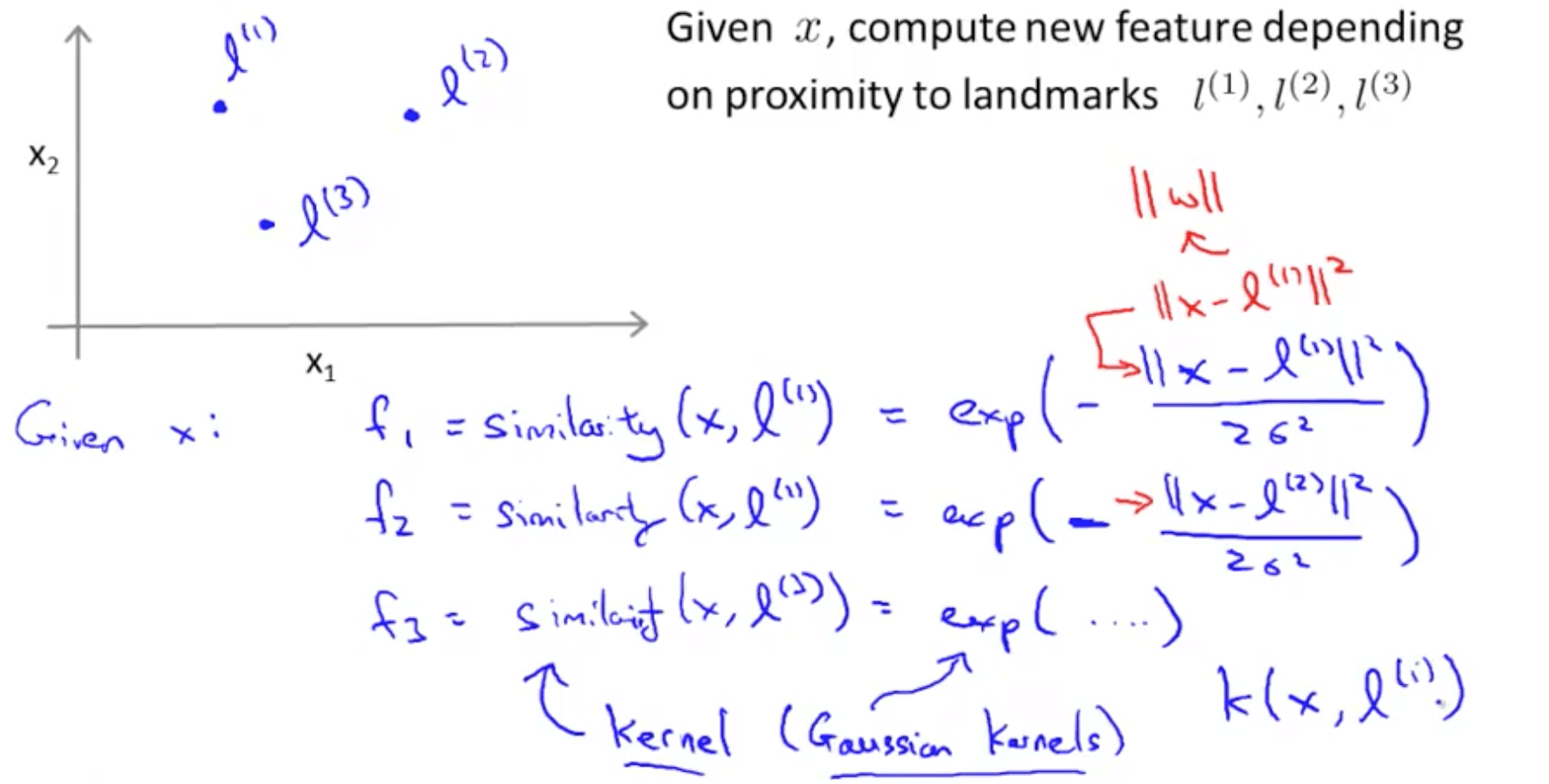• We will manually pick 3 landmarks (points)
• Given an example x, we will define the features as a measure of similarity between x and the landmarks
• f1 = similarity(x, l(1))
• f2 = similarity(x, l(2))
• f3 = similarity(x, l(3))
• The different similarity functions are Gaussian Kernels
• This kernel is often denoted as k(x, l(i))
• Kernels and similarity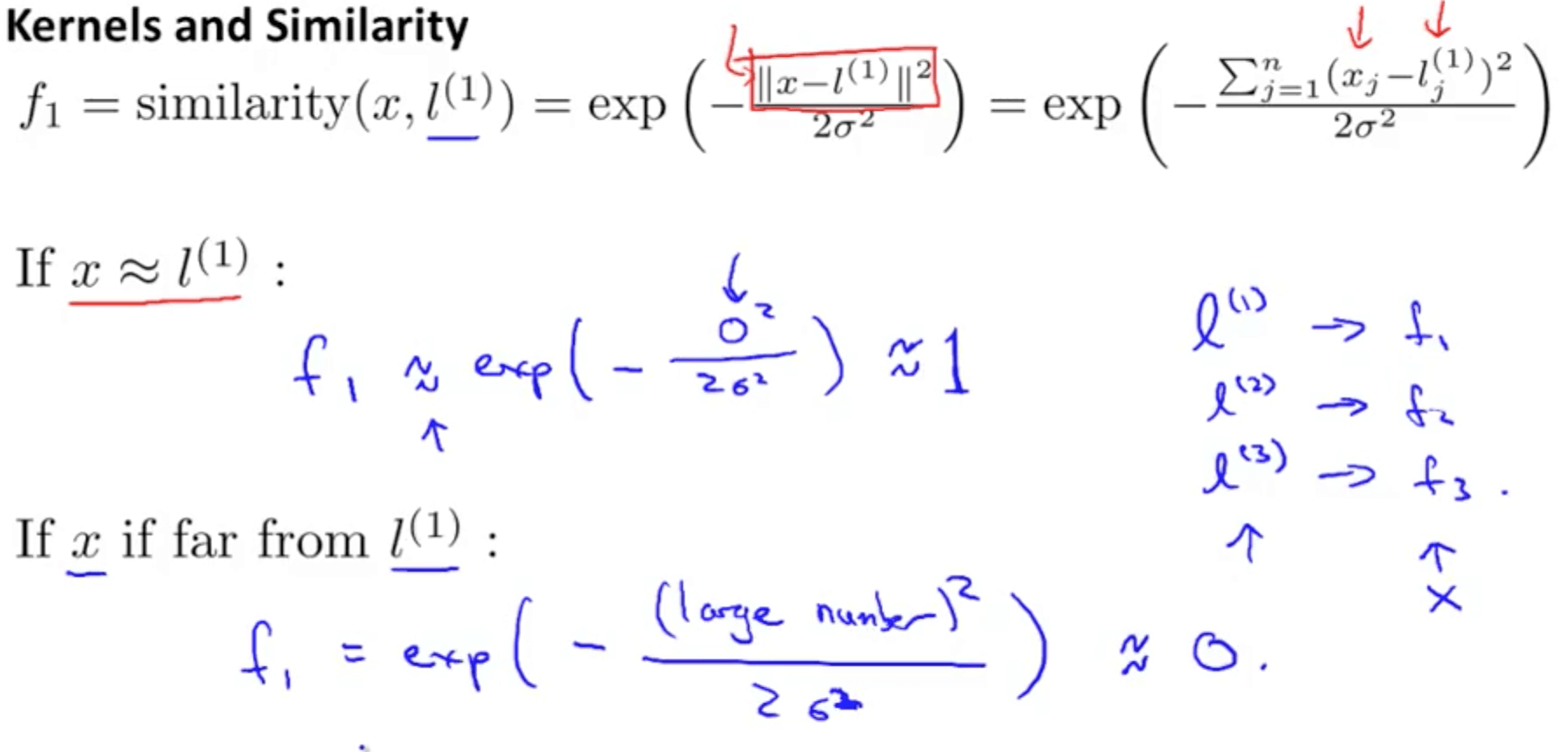• Kernel Example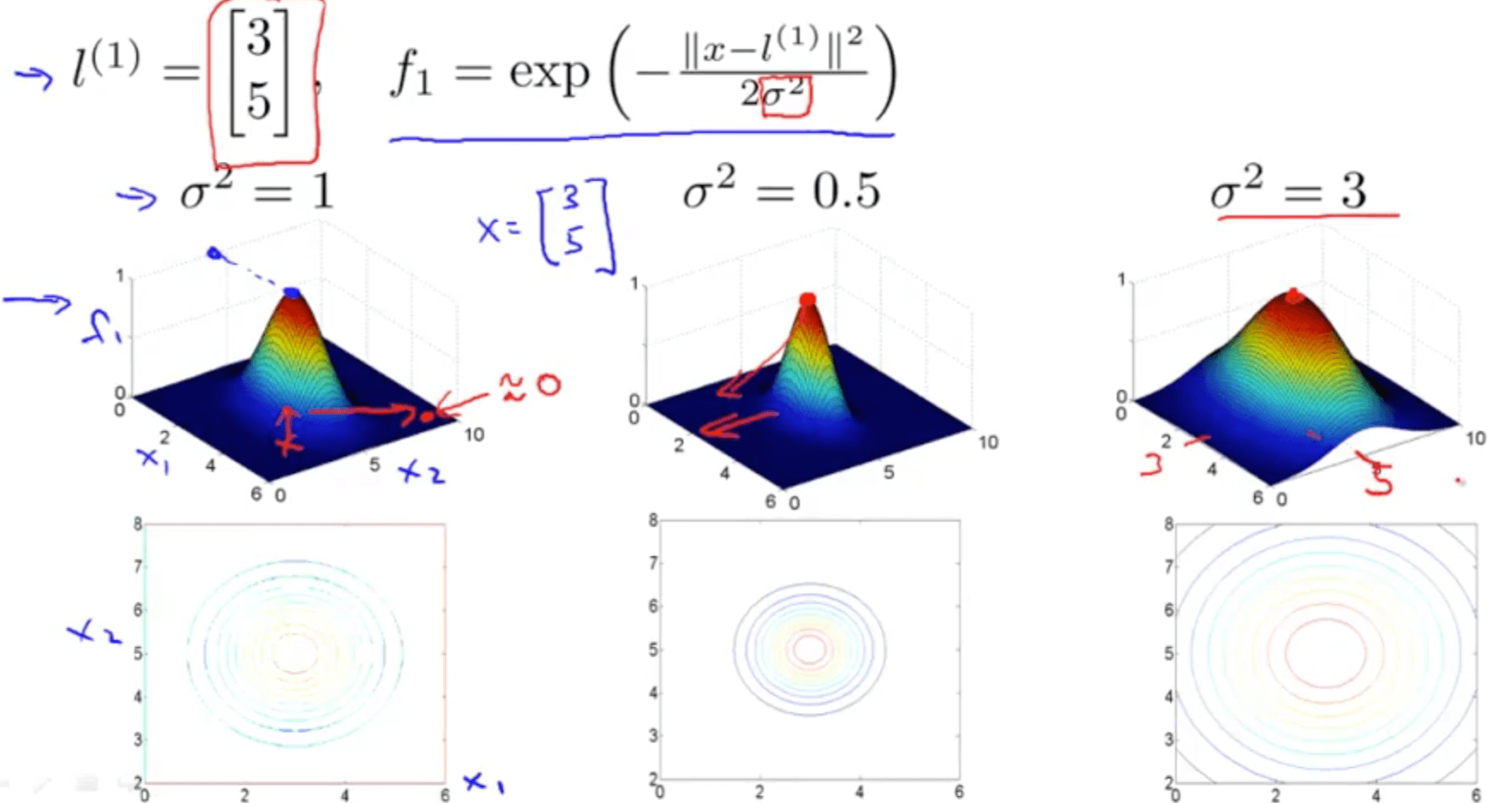• As you increase sigma square
• As you move away from l1, the value of the feature falls away much more slowly
• Kernel Example 2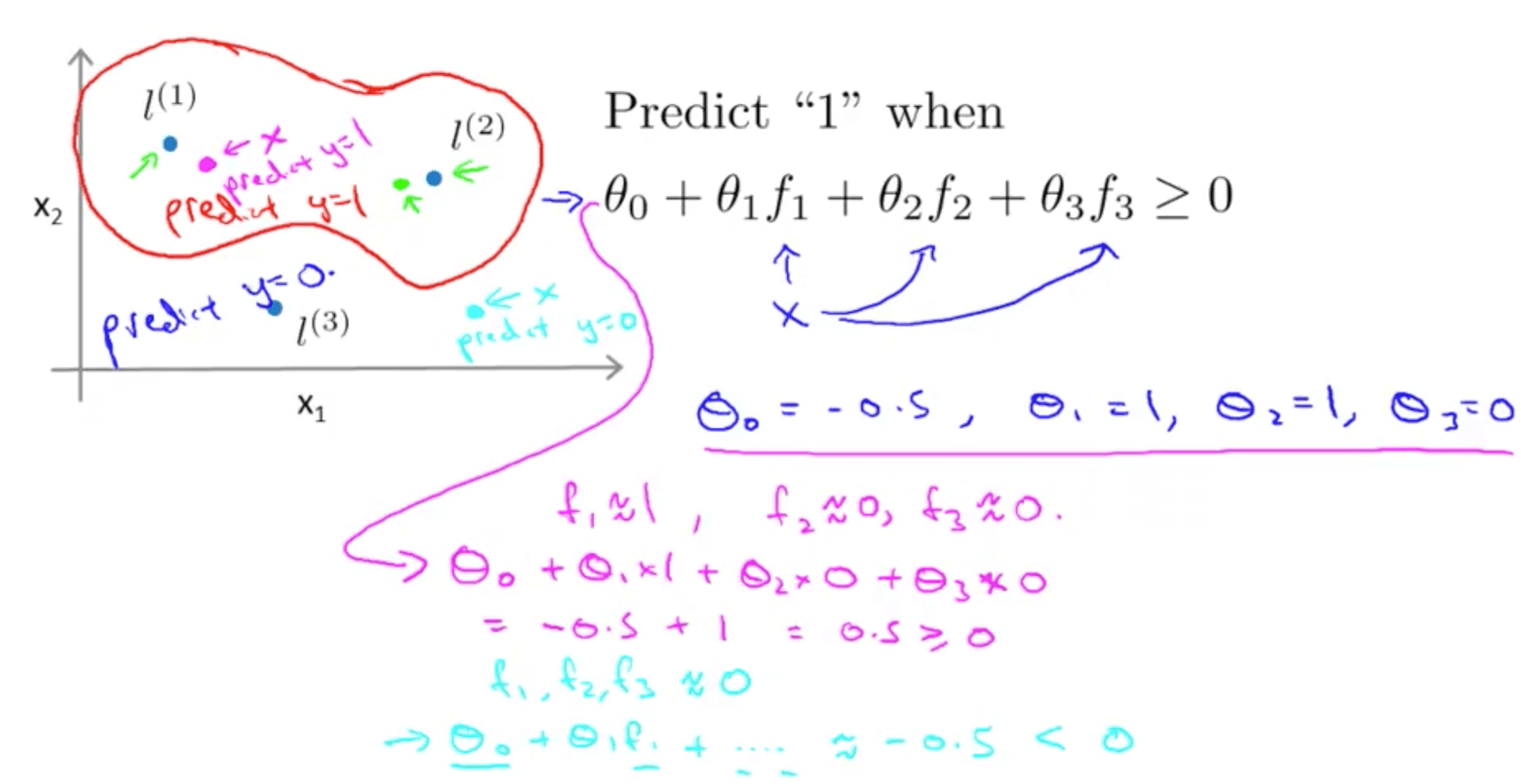• For the first point (magenta), you will predict 1 because hθ >= 0
• For the second point (cyan), you will predict 0 because hθ < 0
• We can learn complex non-linear decision boundaries
• We predict positive when we’re close to the landmarks
• We predict negative when we’re far away from the landmarks
• Questions we have yet to answer
• How do we get these landmarks?
• How do we choose these landmarks?
• What other similarity functions can we use beside the Gaussian kernel?

### 2b. Kernels II

• Choosing the landmarks
• For every training example, we’ll choose the landmarks with the exact locations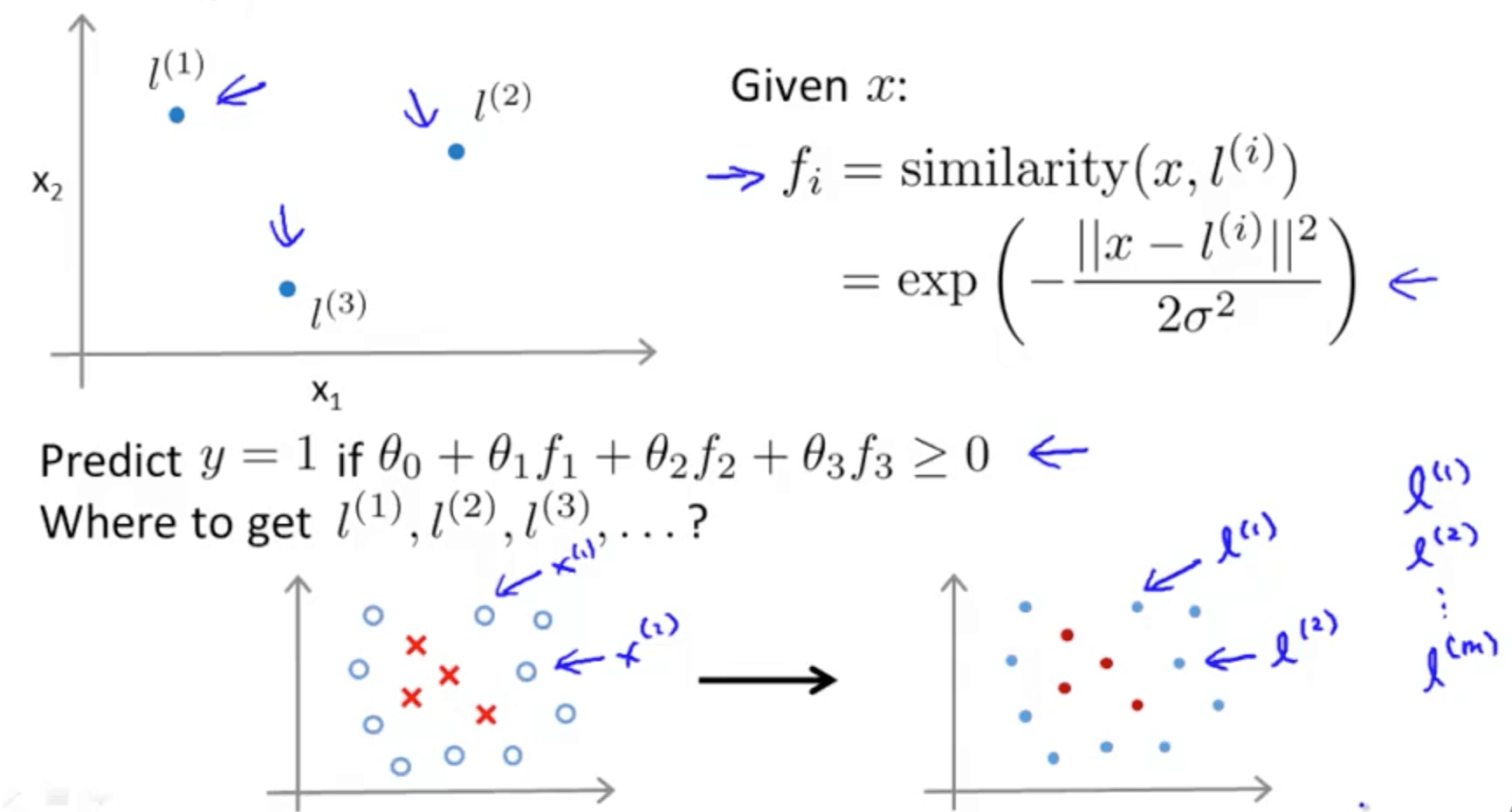• SVM with kernels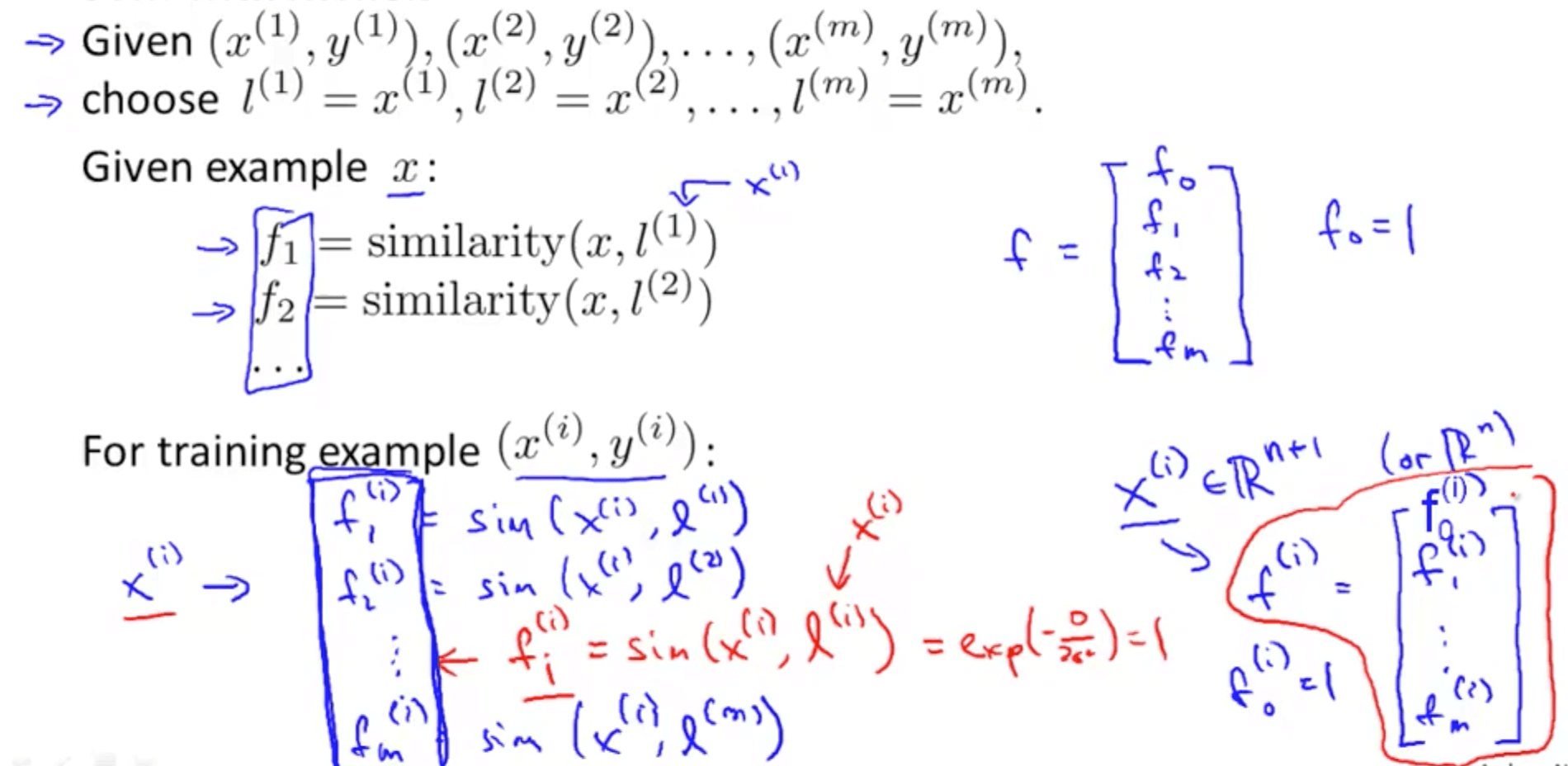• When we solve the following optimization problem, we get the features
• We do not regularize thetaθ, so it starts from 1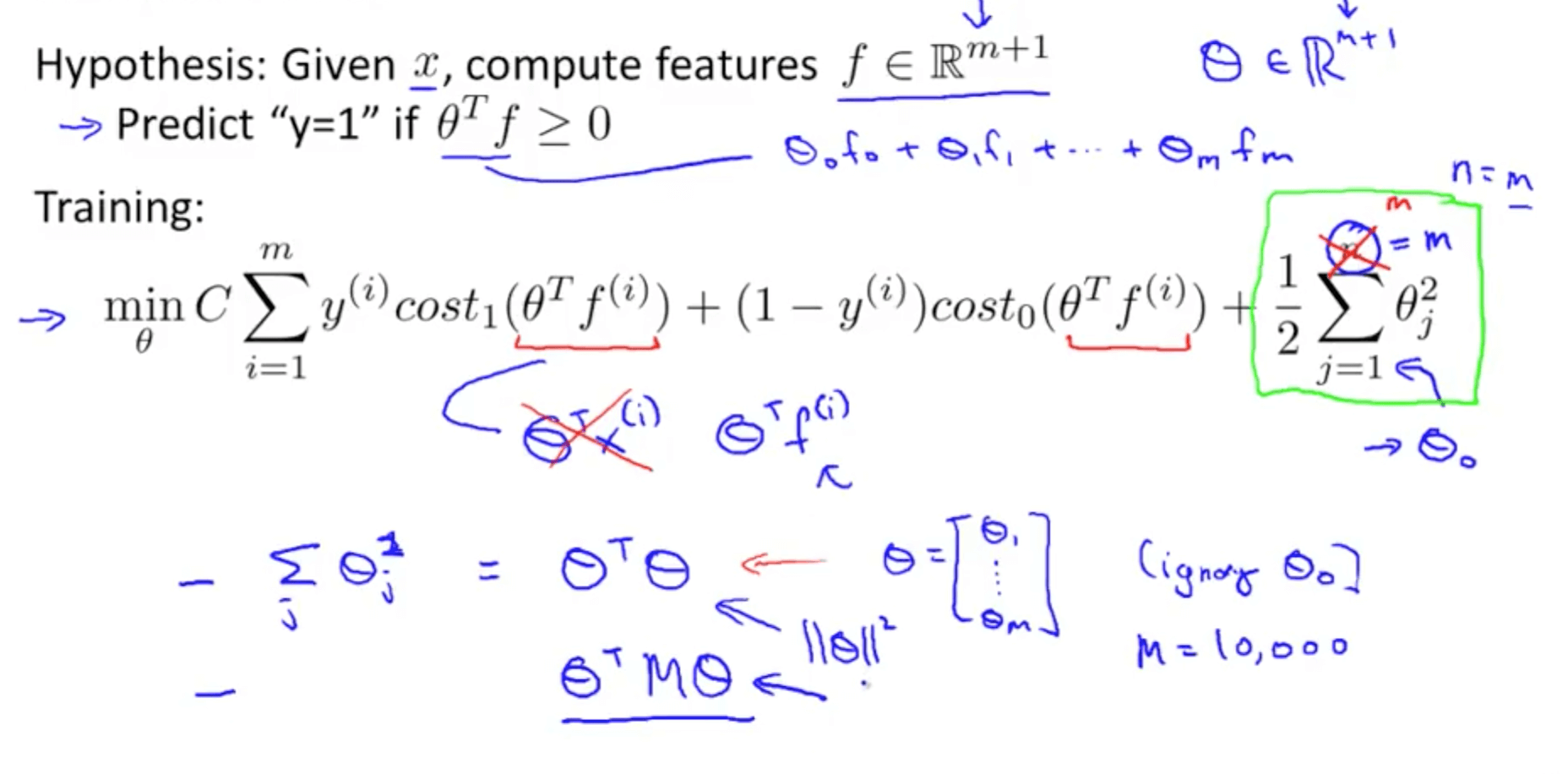• SVM parameters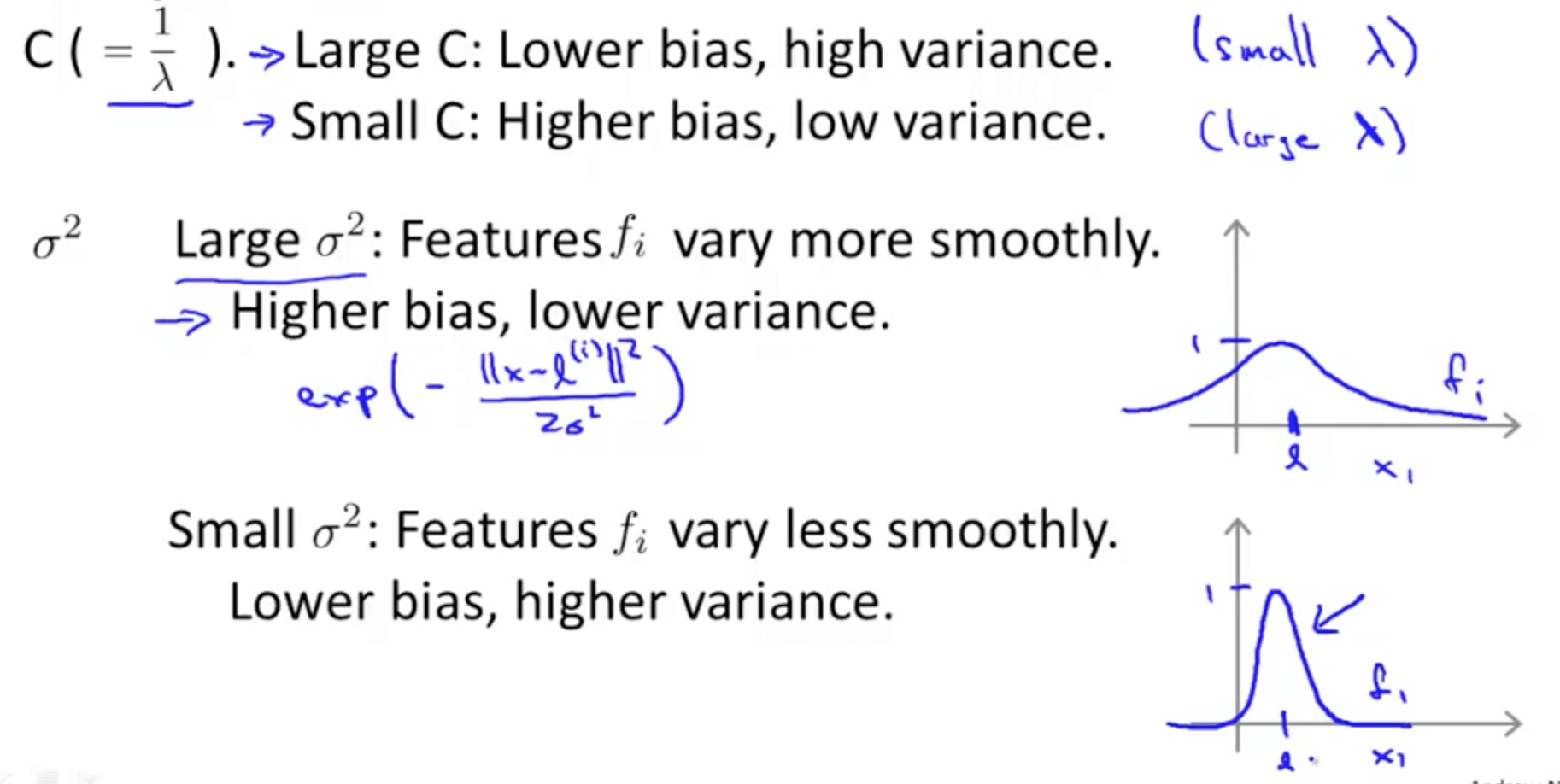## 3. SVMs in Practice

• We would normally use an SVM software package (liblinear, libsvm etc.) to solve for the parameters θ
• You need to specify the following
• Choice of parameter C
• Choice of kernel (similarity function)
1. No kernel is essentially “linear kernel”
• Predict “y = 1” if θ_transpose * x >= 0
• Use this when n is large (number examples) & m is small
2. Gaussian kernel
• For this kernel, we have to choose σ^2
• Use this when n is small (number of examples) and/or m is large
• If you choose a Gaussian kernel
• Octave implementation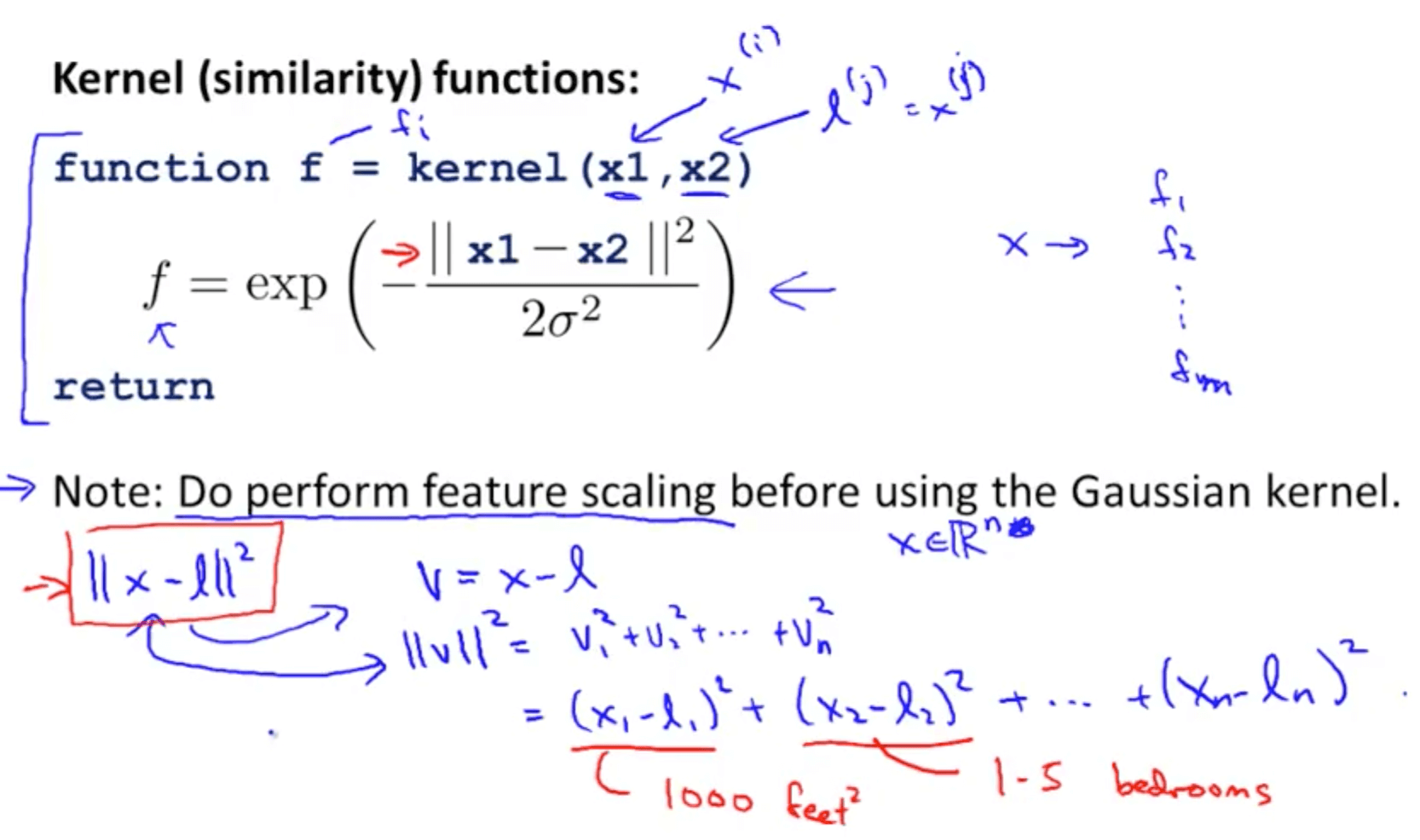• We have to do feature scaling before using Gaussian kernel
• This is because if we don’t, ll x - l ll^2 would be dominated mainly by the features that are large in scale such as the 1000sqft feature
• The Gaussian kernel is also parameterized by a bandwidth pa- rameter, σ, which determines how fast the similarity metric decreases (to 0) as the examples are further apart
• Other choices of kernel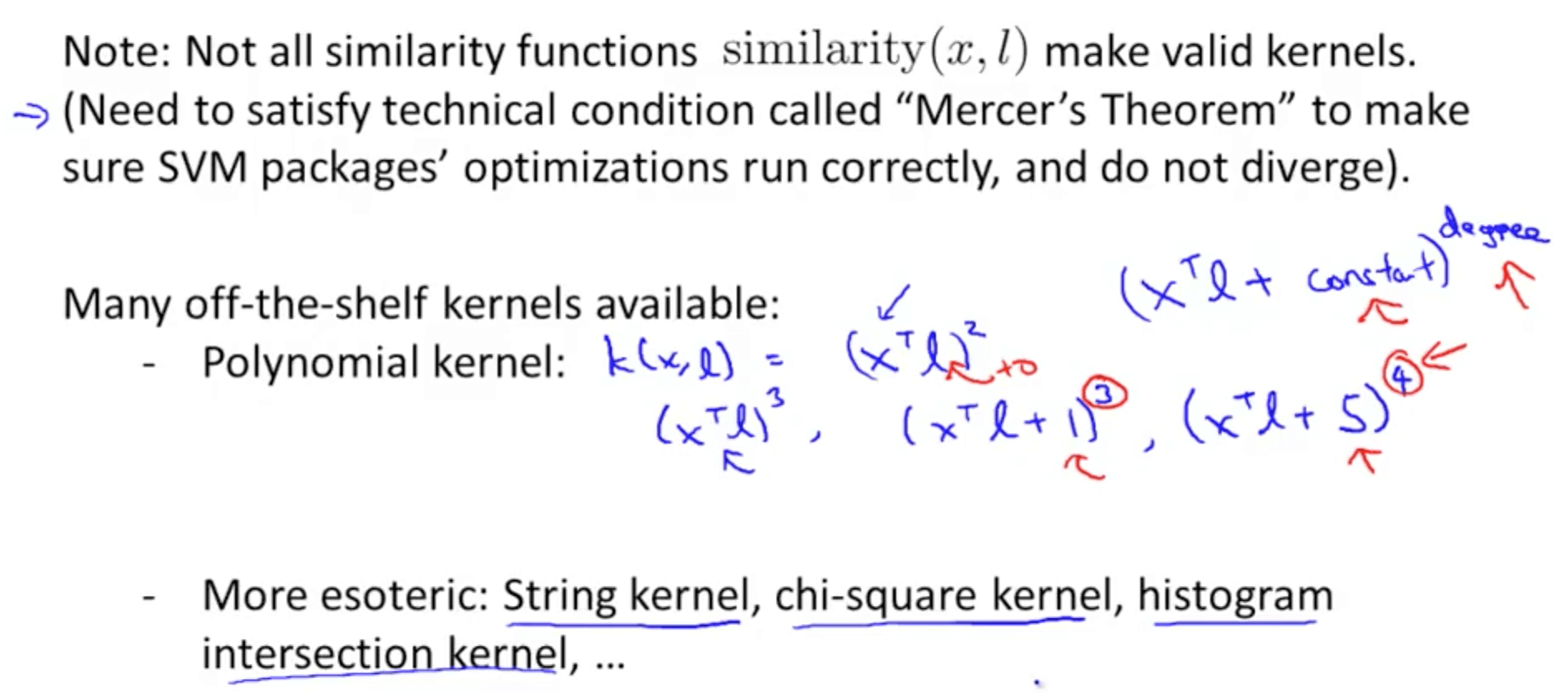• Multi-class classification
• Typically most packages have this function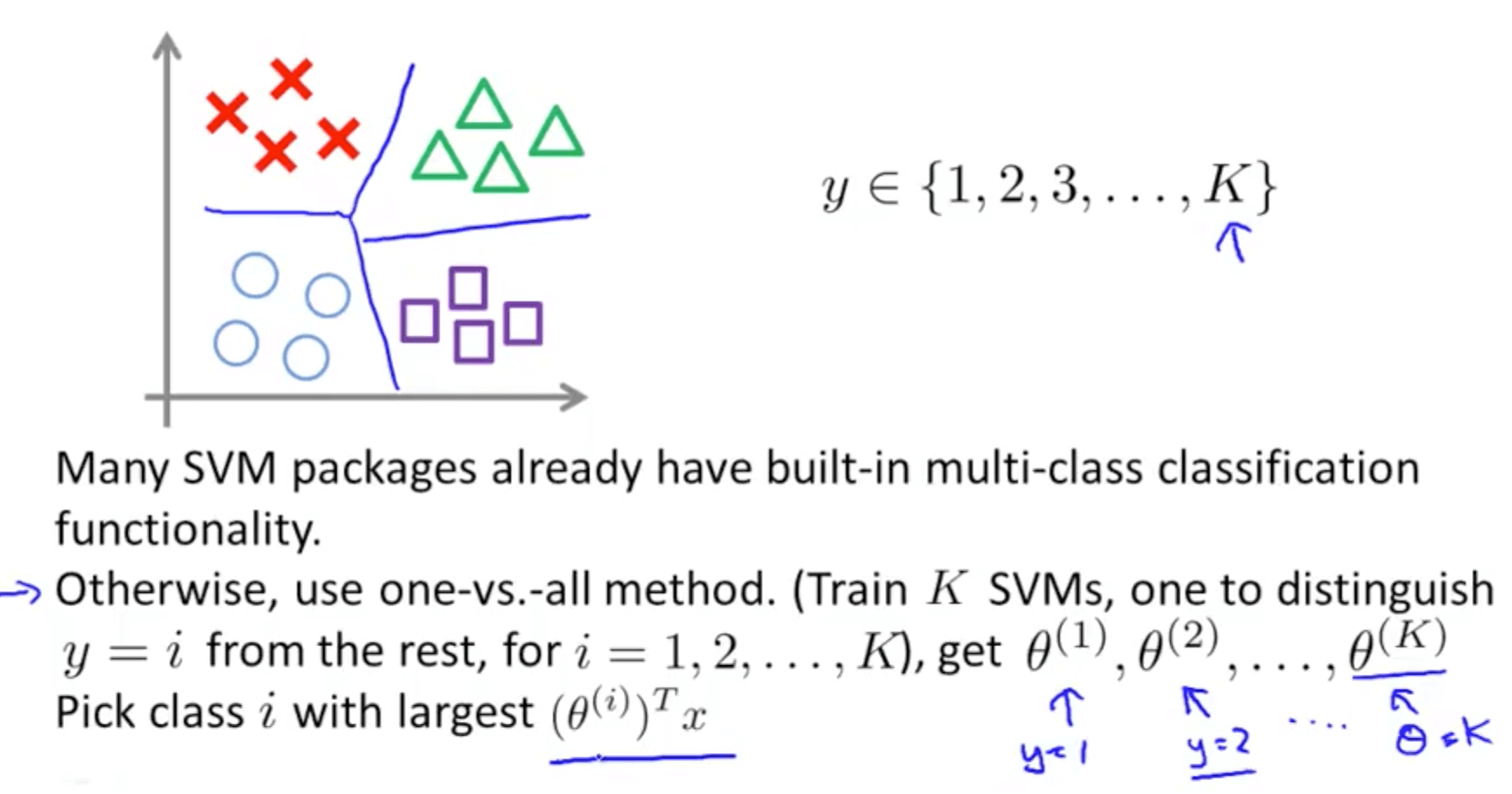• Logistic Regression vs SVMs
• When do we use logistic regression and when do we use SVMs?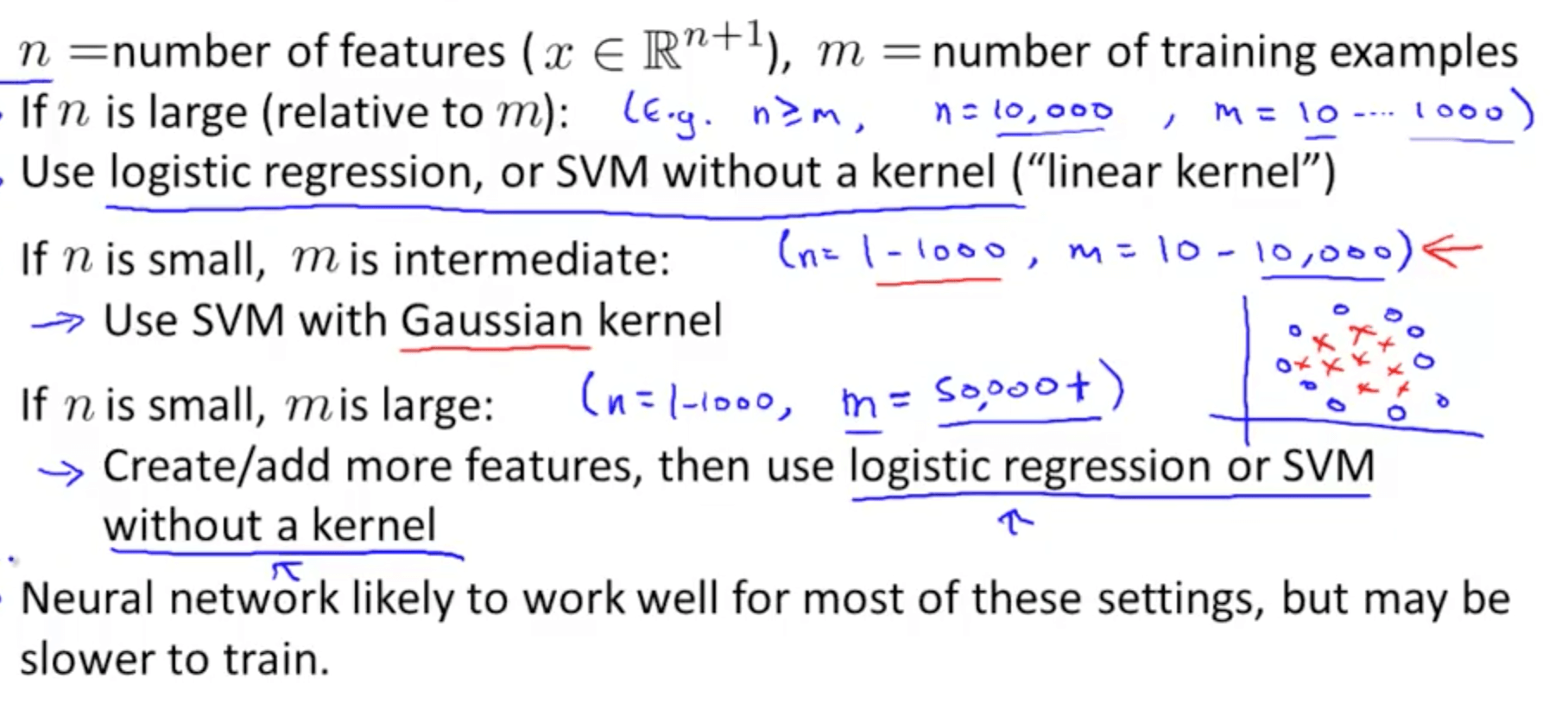• The key thing to note is that if there is a huge number of training examples, a Gaussian kernel takes a long time
• The optimization problem of an SVM is a convex problem, so you will always find the global minimum
• Neural Network: non-convex, may find local optima
Tags: